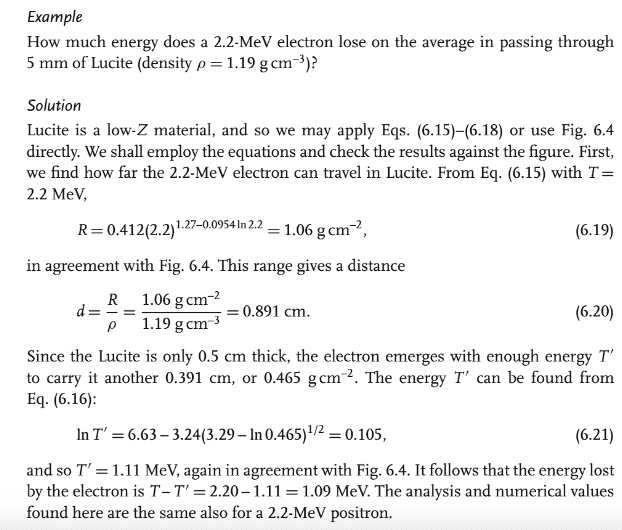# Range of a Beta Particle

## Homework Statement

Q- a positron emerges normally from a 4-mm thick slab of plastic (density= 1.14g/cm^3) with an energy of 1.62 MeV. What is the energy of the particle when it entered the slab?

## Homework Equations

Range when 0< T <= 2.5 MeV: R= .412+T^[1.27-.0954*ln(T)] where T is kinetic energy of beta particle
also ln(T)= 6.63-3.24[3.29-ln(R)]^.5

## The Attempt at a Solution

Ok I know initial slab is 4mm or .4 cm and has a density of 1.14 g/cm^3. thus it has a range of .456 g/cm^2

now the range of the beta particle after it left the slab with E= 1.62 MeV is:

R= .412*1.62^[1.27 - .0954*ln(1.62)]= .744

now range total is just: R_total = .456 + .744 = 1.2

now we can solve what the initial T was going into the slab via:

ln(T) = 6.63-3.24[3.29-ln(1.2)]^.5= .9183
thus T= e^(.9183) = 2.5 MeV

However I should be getting 2.2 MeV. Does anyone see where I am going wrong?

Hi,
for me it is not a problem of beta range in matter.
In the problem you have not a beta Spectrum and you must calculate the Energy lost in platic.

Hi,
for me it is not a problem of beta range in matter.
In the problem you have not a beta Spectrum and you must calculate the Energy lost in platic.

This is a single mono-energetic particle. Shouldn't need a beta spectrum.

"This is a single mono-energetic particle. Shouldn't need a beta spectrum. "
it is a question or an affirmation ?

Affirmation. I know I am not suppose to use a beta spectrum here. I may be starting to convince myself 2.2 from the back of the book is actually wrong

but it is not a problem of beta range in matter.
you must calculate the Energy lost in platic of a mono-energetic positrion.

RPinPA
Homework Helper
I'm trying to follow your calculations to check them but having a great deal of difficulty. Can you explain these equations a little more? For instance:
Range when 0< T <= 2.5 MeV: R= .412+T^[1.27-.0954*ln(T)] where T is kinetic energy of beta particle
What is this equation? Range in what medium?

also ln(T)= 6.63-3.24[3.29-ln(R)]^.5
Here is an entirely different relationship between T and R. At a glance, they are not the same relationship. So why are you saying that both of these relationships between T and R are applicable? What is this one? Under what assumptions?

Ok I know initial slab is 4mm or .4 cm and has a density of 1.14 g/cm^3. thus it has a range of .456 g/cm^2
Thus, WHAT has a range of 0.456 g/cm^2? And how is "g/cm^2" a range? A range is a distance probably in meters. What formula did you use to calculate this quantity?

Oh wait. Is that the product of those two things? So that's the number of grams per cm^2 cross section of the slab? How is that relevant? The particle only goes through one point, cross-section area doesn't come into it in any way, shape or form.

You're trying to find out how much energy was lost in passing through the slab. I don't see any "relevant equation" you provided where the material properties are related to energy. So what exactly did go into your calculation and what equation did you use that took the density of the material into account?

This is the range of a beta particle in any medium that has an effective Z of <10

Also that second relationship is given to me. See image.

The assumption we are making in this problem is that the electron will travel in a straight line through the medium. I know this isn't true at all but for the purposes of this problem that is what they want us to do. Therefore with that assumption we can use the range of the beta particle to give us an approximation how far the particle will travel. I attached an example of a similar type of calculation from the book as well.#### Attachments

gneill
Mentor
I may be starting to convince myself 2.2 from the back of the book is actually wrong
I suspect that you're right.

Why don't you try plugging 2.2 MeV as the starting energy and work the example you posted above in exactly the same way that they did (using your density and thickness of course) and see if you can arrive at a 1.62 MeV positron exiting the slab?

•Binder12345
I suspect that you're right.

Why don't you try plugging 2.2 MeV as the starting energy and work the example you posted above in exactly the same way that they did (using your density and thickness of course) and see if you can arrive at a 1.62 MeV positron exiting the slab?
Thank you!

RPinPA
•